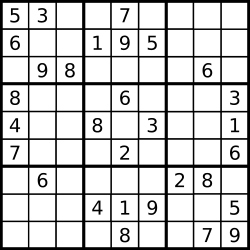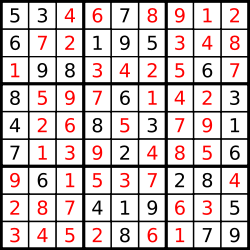## Sudoku Solver

06/25/2016 Hash Table Backtracking

## Question

Write a program to solve a Sudoku puzzle by filling the empty cells.

Empty cells are indicated by the character '.'.

You may assume that there will be only one unique solution.A sudoku puzzle……and its solution numbers marked in red.

## Solution

Result: Accepted Time: 4ms

Here should be some explanations.


bool row,col,rco;
int blank,cnt = 0;
bool dfs(int lev,char ** sudo,const int rsz,const int csz)
{
if(lev >= cnt)
return true;
int x  = blank[lev],y = blank[lev];
for(int i= 1; i < 10; i++)
if(!row[x][i] && !col[y][i] && !rco[x/3][y/3][i])
{
sudo[x][y] = '0' + i;
row[x][i] = col[y][i]  = rco[x/3][y/3][i] = true;
if(dfs(lev+1,sudo,rsz,csz))
return true;
row[x][i] = col[y][i]  = rco[x/3][y/3][i] = false;
}
return false;
}

void solveSudoku(char** board, int rsz, int csz)
{
cnt = 0;
memset(row,0,sizeof(row));
memset(col,0,sizeof(col));
memset(rco,0,sizeof(rco));
for(int r = 0; r < rsz; r++)
for(int c = 0,tmp; c < csz; c++)
if(isdigit(tmp=board[r][c]))
row[r][tmp-'0'] = col[c][tmp - '0'] = rco[r/3][c/3][tmp -'0'] =  true;
else
{
blank[cnt] = r;
blank[cnt++] = c;
}
dfs(0,board,rsz,csz);
}


Complexity Analytics

• Time Complexity: $O(n!)$?
• Space Complexity: $O(1)$